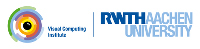OpenMesh
Using mesh attributes and traits

this example shows how to change the data type for positions, normals, colors, and texture,

In the previous tutorial (Using standard properties) we learned to use standard properties by calling the appropriate request method. Unlike the custom properties, where the user specifies the data type by passing the type to the handle (e.g. MyMesh::FPropHandleT< int>), the data types of the standard properties are defined by so-called mesh traits. With traits we can customize and extend the mesh data structure. We can do this by changing two important features

1. changing data type for positions, normals, colors, and texture coordinates
2. extend mesh entities Vertex, Face, Edge, and Halfedge (see tutorial 'Extending the mesh using traits')

Let's start. Every custom traits should derive from the default traits

As mentioned, we can change the basic data types for the basic types MyMesh::Point, MyMesh::Normal, MyMesh::Color, and MyMesh::TexCoord. We can use the provided vector class or we use a different one from another library. Here we simply replace the default type OpenMesh::Vec3f (defined in the OpenMesh::DefaultTraits) for positions and normals with OpenMesh::Vec3d

typedef OpenMesh::Vec3d Point;

(In general it's better to have the same scalar type for the point and normal vector, for instance double in this case. Otherwise we have to cast quite a lot depending on the implementation of the vector class.)

Be aware that these settings overwrite the ones of the parent traits class! As we usually derive from the DefaultTraits let's have a close look.

Actually the struct OpenMesh::DefaultTraits is merely empty. It solely defines the types for Point, Normal, TexCoord, and Color and one attribute, that we used implicitly all the time:

// HalfedgeAttributes( OpenMesh::Attributes::PrevHalfedge );

The attribute PrevHalfedge is different, as it does not control a property. Yet it has a great impact on the resulting mesh type, as it adds additional information to the halfedge structure. The impact is twofold:

2. increase of memory consumption

Using this feature depends highly on our needs. One situation where the previous halfedges are quite handy, is the mesh member function add_face(). The execution time for the member function drops dramatically, when the information about the previous halfedge is available. Usually we want to have this information. But if not, because we must save memory, we can easily remove it with

// HalfedgeAttributes( OpenMesh::Attributes::None );

Then we need 8 bytes less per edge, which can be quite a lot as one can derive from the Euler formula (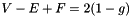), that for a regular triangle meshes with genus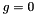the number of edges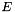is approximately three times the number of vertices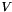: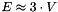.

The complete source looks like this:

#include <iostream>
#include <typeinfo>
// --------------------
#include <OpenMesh/Core/IO/MeshIO.hh>
#include <OpenMesh/Core/Mesh/TriMesh_ArrayKernelT.hh>
#include <OpenMesh/Core/Geometry/VectorT.hh>
#ifndef DOXY_IGNORE_THIS
// Define my personal traits
{
// Let Point and Normal be a vector of doubles
typedef OpenMesh::Vec3d Point;
typedef OpenMesh::Vec3d Normal;
// HalfedgeAttributes( OpenMesh::Attributes::PrevHalfedge );
// Uncomment next line to disable attribute PrevHalfedge
// HalfedgeAttributes( OpenMesh::Attributes::None );
//
// or
//
// HalfedgeAttributes( 0 );
};
#endif
// Define my mesh with the new traits!
// ------------------------------------------------------------------ main ----
int main(int argc, char **argv)
{
MyMesh mesh;
if (argc!=2)
{
std::cerr << "Usage: " << argv << " <input>\n";
return 1;
}
// Just make sure that point element type is double
!= typeid(double) )
{
std::cerr << "Ouch! ERROR! Data type is wrong!\n";
return 1;
}
// Make sure that normal element type is double
!= typeid(double) )
{
std::cerr << "Ouch! ERROR! Data type is wrong!\n";
return 1;
}
// Add vertex normals as default property (ref. previous tutorial)
mesh.request_vertex_normals();
// Add face normals as default property
mesh.request_face_normals();
{
std::cerr << "Error loading mesh from file " << argv << std::endl;
return 1;
}
// If the file did not provide vertex normals, then calculate them
mesh.has_face_normals() && mesh.has_vertex_normals() )
{
// let the mesh update the normals
}
// move all vertices one unit length along it's normal direction
for (MyMesh::VertexIter v_it = mesh.vertices_begin();
v_it != mesh.vertices_end(); ++v_it)
{
std::cout << "Vertex #" << *v_it << ": " << mesh.point( *v_it );
mesh.set_point( *v_it, mesh.point(*v_it)+mesh.normal(*v_it) );
std::cout << " moved to " << mesh.point( *v_it ) << std::endl;
}
return 0;
}

Project OpenMesh, ©  Computer Graphics Group, RWTH Aachen. Documentation generated using doxygen .